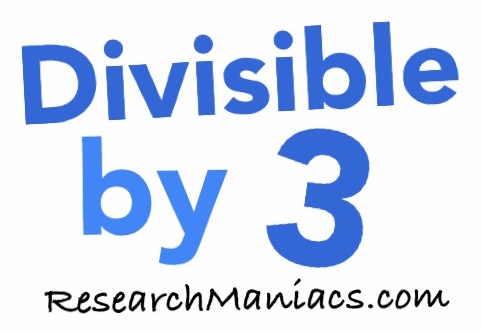Is 314 divisible by 3?Is 314 divisible by 3? In other words, if you divide 314 by 3, will you get a whole number with no remainder?

Of course, you could use a calculator to find out if 314 is divisible by 3, but what fun would that be?

To find out if 314 is divisible by 3, we will add up the numbers that make 314 as follows: 3 + 1 + 4 = 8

We know that if the sum of the numbers that make up 314 is divisible by 3, then 314 is divisible by 3.

Since the sum of the digits in 314 is not divisible by 3, 314 is also NOT divisible by 3. Thus, the answer to the question "Is 314 divisible by 3?" is as follows:

No

Note: If you divide 314 by 3, you get 104.67 which is not a whole number.

Divisible by 3?
Do you need to check another number? Enter a number below to see if it is divisible by 3.

Is divisible by 3?

Is 315 divisible by 3?
Can you do it on your own now? If not, go here for the next number on our list.